# Hydraulic Pumps## Different hydraulic pumps

The hydraulic pump - a key element in the hydraulic system. Your task is to make a choice!Hydraulic pump animation

Hydraulic pumps have to press a fluid (usually oil) into the working circuit. Criteria for selecting a pump here are mainly:

• Required operating pressure
• Flow rate
• Constant flow rate or adjustable flow rate, possibly with two directions
• Noise

Hydraulic pumps can be broadly classified into: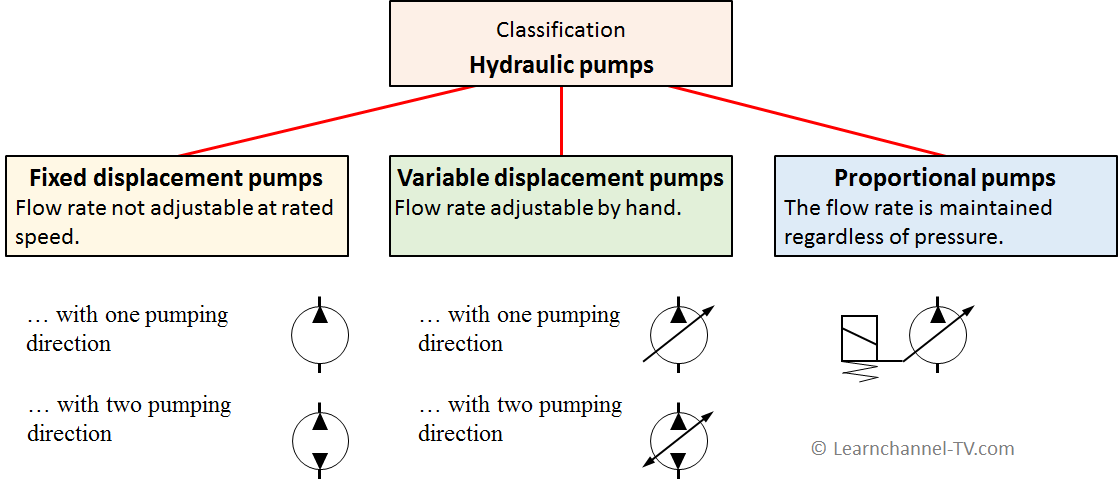Classification Hydraulic Pumps

### How to determine the effiency of a pump

Pumps usually convert electrical energy into hydraulic energy. Of course, energy conversion is always associated with loss. There are three categories of effiency to describe hydraulic pumps: Volumetric effiency, mechanical/hydraulic effiency and the overall effiency.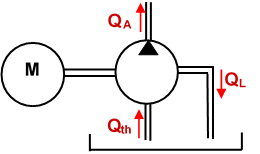Volumetric efficiency of a hydraulic pump

#### Volumetric efficiency ηVol

The Volumetric efficiency is determined by dividing the actual flow delivered by a pump at a certain pressureby its theoretical flow.

The design features piston diameter, piston stroke and piston number determine the theoretical delivery volume Vth for one revolution. Furthermore the speed of the drive as a further parameter determines the theoretical volume flow Qth of the pump:  QTh = Speed (rpm)   *   Displacement Vth (cc/rev)

The Actual flow should be measured using a flow meter. So you can define the Volumetric Efficiency ηVol as in the following:

ηVol =   QA  /  Qth         with QA = Qth   -   QL

Vth  = theoretical volume delivered at one pump revolution
QA  = Actual flow rate
QL  = Internal Leakage Flow of the pump
Qth = Theoretical flow rate
ηVol = Volumetric efficiency

The volumetric efficiency can also be used to determine the condition of a hydraulic pump, based on its increase in internal leckage through wear or damage! But without reference to theoretical flow, the actual flow measured by the flow meter would be meaningless.

#### Mechanical efficiency (due to friction losses)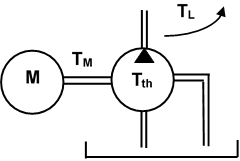Mechanical efficiency of a hydraulic pump

Since a pump is a mechanical component, mechanical friction losses also occur. Therefore, the torque actually required must be correspondingly greater than the theoretically calculated torque to drive the hydraulic pump:

TM =   Tth   +  TL

One can thus define a mechanical efficiency:       ηmech  =   Tth / TA

TA  = Actual torque delivered to the pump
Tth  = Theoretical Torque required to operate the pump
Qth = Theoretical flow rate
TL = Torque losses due to friction

#### Overall efficiency of the pump

Overall efficiency is used to calculate the drive power required by a pump at a given flow and pressure. In the field of automation the pump is usually driven by an electric drive. Due to the leakage losses and the mechanical losses PL of the pump, the delivered hydraulic power P2 must be less than the input power P1.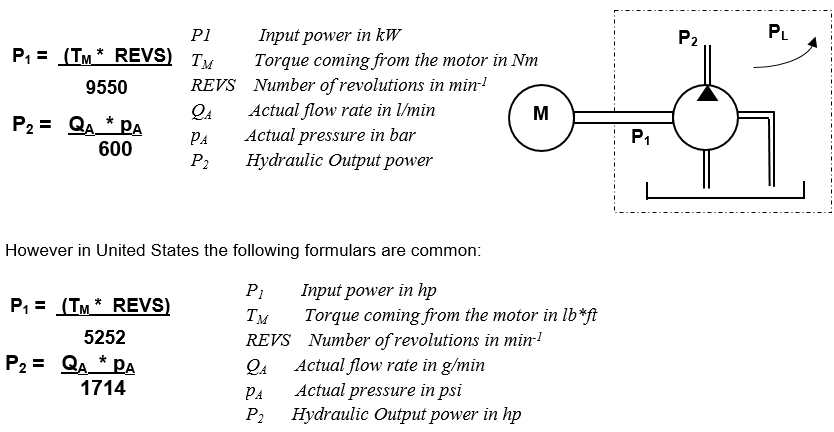Overall efficiency of a hydraulic pump

This results in the Overall Efficiency ηO of the pump:  ηO =   P2 / P1       or    ηO =   ηmech  *   ηvol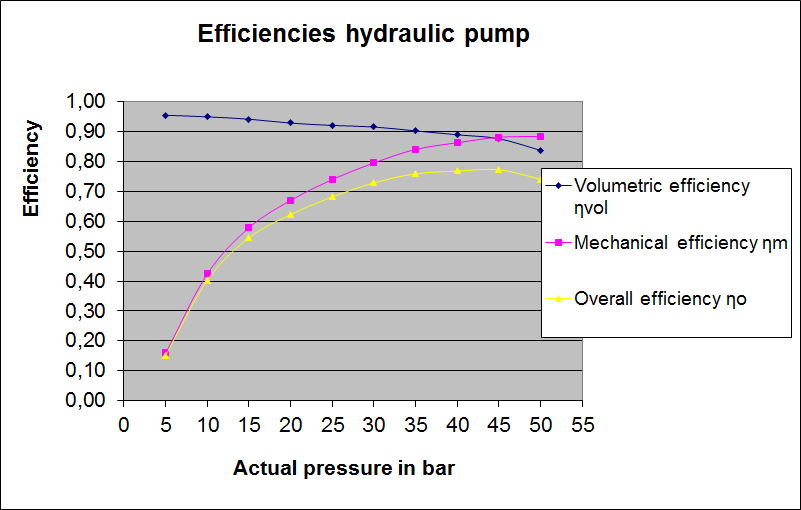Chart - Efficiency Hydraulic Pumps

### Power flow of a hydraulic system

With the help of a specific flow diagram, called Sankey diagram, the losses in a hydraulic system can be shown in a simple way: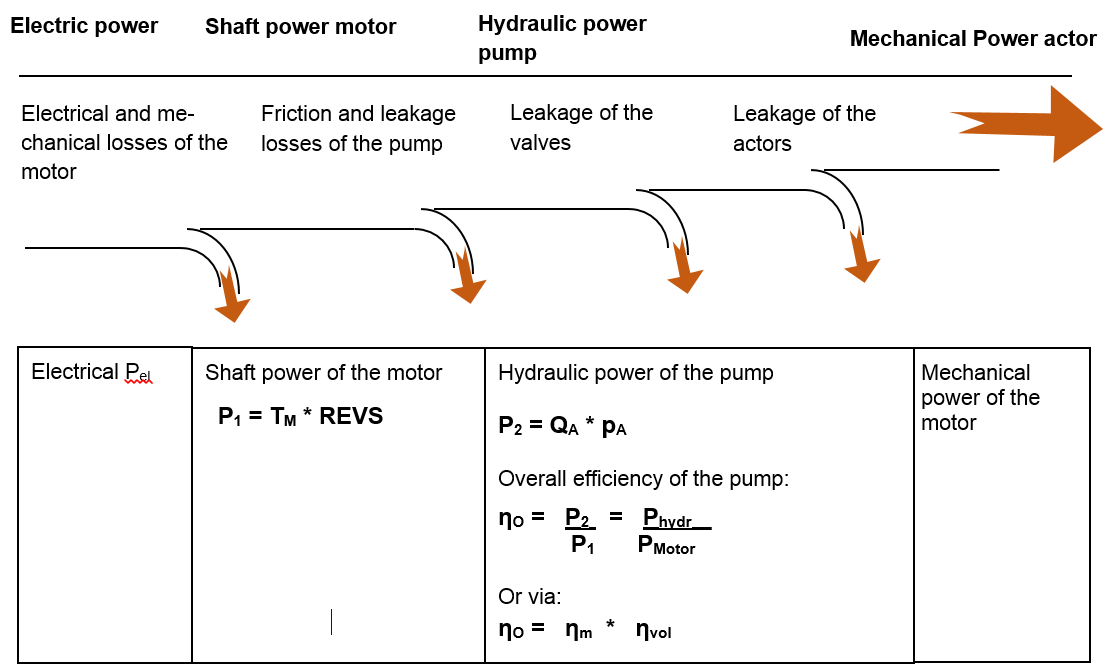Power Flow of a hydraulic system

### Work order: Determe the efficiencies of a hydraulic pump

Fill in the missing information of the gear pump within the table and record the characteristic curves for the mechanical, volumetric and total efficiency.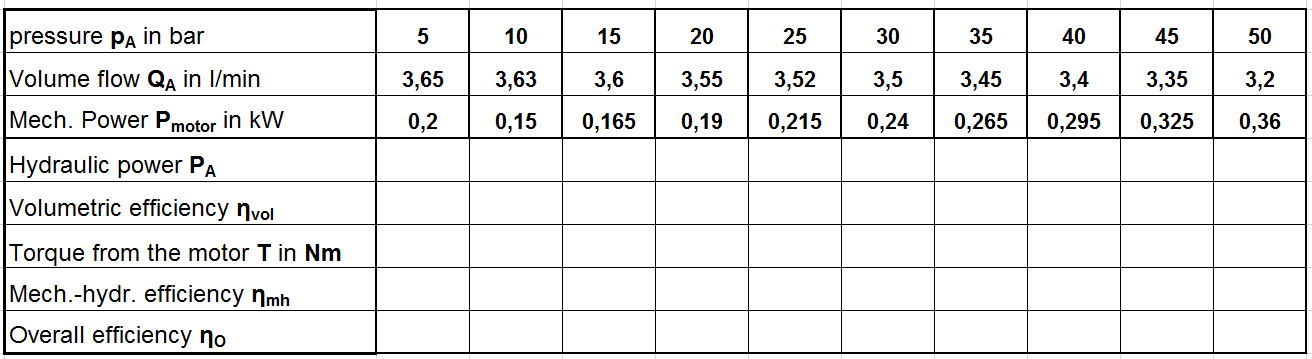Work order calculate efficiencies hydraulic pump

Use the following link to download the Excel spreadsheet to calculate the different efficiencies:

Hydraulic Pump efficiency curves